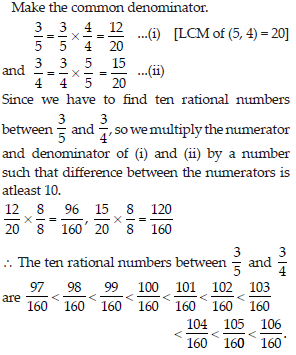# Rational Numbers (Mathematics) Class 8 - NCERT Questions

Q 1.

Using appropriate properties, find.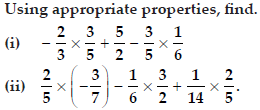SOLUTION: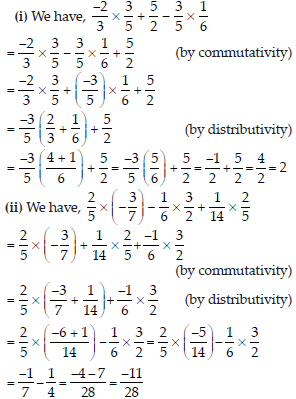Q 2.

Write the additive inverse of each of the following.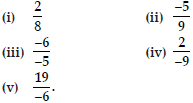SOLUTION: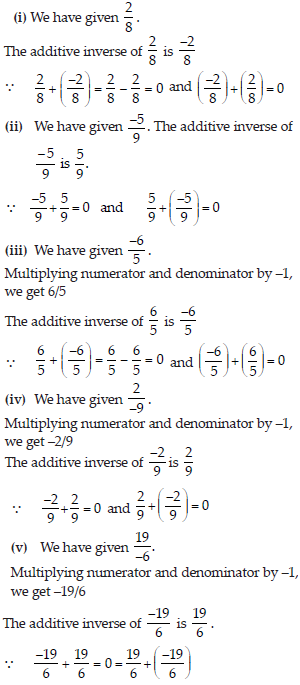Q 3.

Verify that –(–x) = x for
(i) x = 11/15 (ii) x = −13/17.

SOLUTION: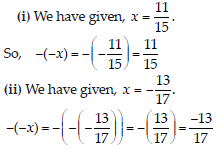Q 4.

Find the multiplicative inverse of the following.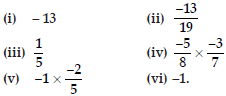SOLUTION: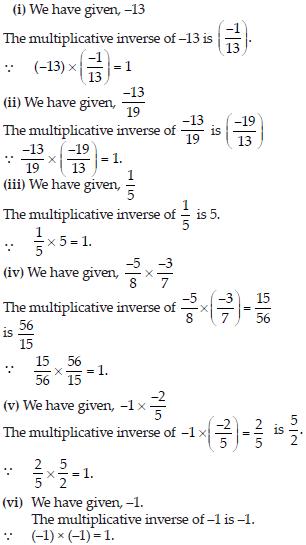Q 5.

Name the property under multiplication used in each of the following.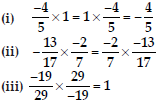SOLUTION: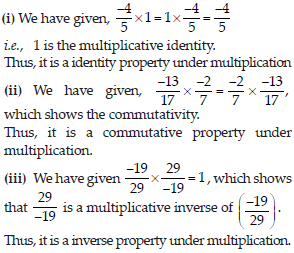Q 6.

Multiply 6/13 by the reciprocal of −7/16

SOLUTION: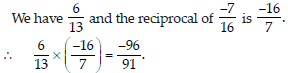Q 7.

Tell what property allows you to compute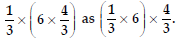SOLUTION: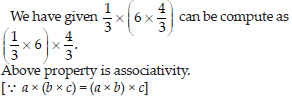Q 8.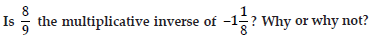SOLUTION: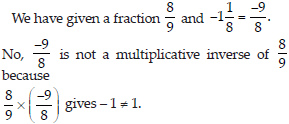Q 9.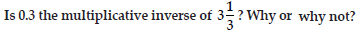SOLUTION: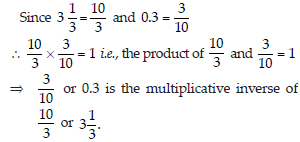Q 10.

Write.
(i) The rational number that does not have a reciprocal.
(ii) The rational numbers that are equal to their reciprocals.
(iii) The rational number that is equal to its negative.

SOLUTION:

(i) 0 is the rational number, which does not have a reciprocal.
(ii) 1 and (–1) are the rational numbers, that are equal to their reciprocals.
(iii) 0 is the rational number that is equal to its negative.

Q 11.

Fill in the blanks.
(i) Zero has ........... reciprocal.
(ii) The numbers ......... and ......... are their own reciprocals.
(iii) The reciprocals of –5 is ............. .
(iv) Reciprocal of 1x, where x ≠ 0 is ......... .
(v) The product of two rational numbers is always a ......... .
(vi) The reciprocal of positive rational number is ........... .

SOLUTION:

(i) Zero have no reciprocal.
(ii) The numbers 1 and –1 are their own reciprocals.
(iii) The reciprocal of –5 is −1/5.
(iv) Reciprocal of 1/x, where x ≠ 0 is x.
(v) The product of two rational numbers is always a rational number.
(vi) The reciprocal of a positive rational number is positive.

Q 12.

Represent these numbers on the number line.
(i) 7/4 (ii) −5/6

SOLUTION: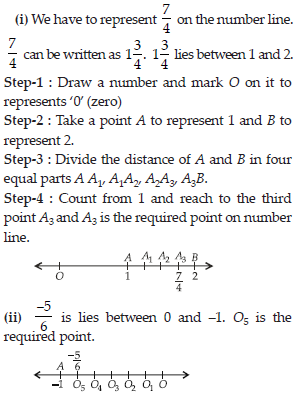Q 13.

Represent −2/11,5/11,9/11 on the number line.

SOLUTION: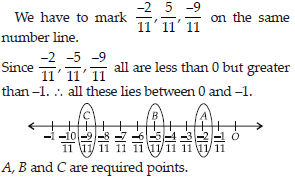Q 14.

Write five rational numbers which are smaller than 2.

SOLUTION: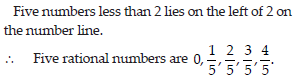Q 15.

Find ten rational numbers between −2/5 and 1/2.

SOLUTION: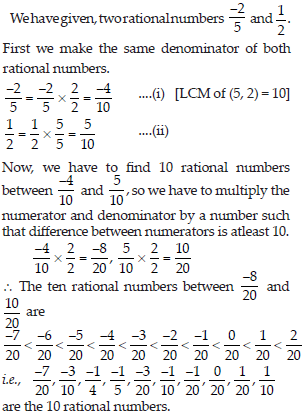Q 16.

Find five rational numbers between

SOLUTION: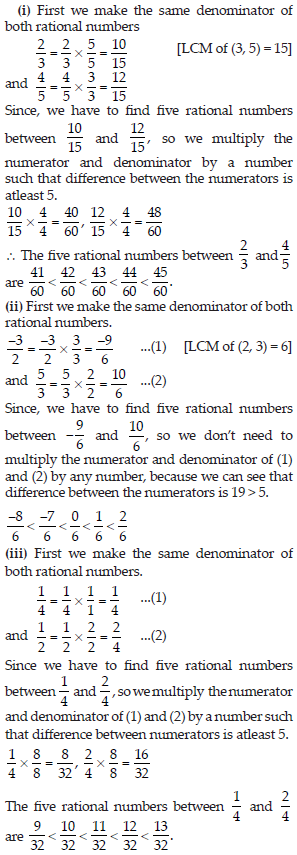Q 17.

Write five rational numbers greater than –2.

SOLUTION: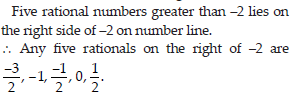Q 18.

Find ten rational numbers between 3/5 and 3/4.

SOLUTION: